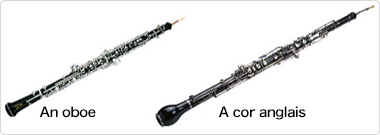# TriviaThis is how the oboe and the cor anglais differ

The oboe is a C woodwind with a base pitch tuned to C, while the cor anglais is an F woodwind with a base pitch tuned a perfect fifth lower, to F. How might the oboe and the cor anglais differ from one another structurally?
There is a law that every doubling of the length of the pipe lowers the pitch by one octave. Thus, to lower the pitch from a C to an F (that is, lowered by a perfect fifth), the length of pipe should be 1.5 times as long.
There is also a law of woodwind instrument design by which a doubling of the length of the pipe also doubles the cross-sectional area of the bore. The cross-section is a circle, so the cross-sectional area can be calculated as the radius × the radius × π (the ratio of the circumference of a circle and its diameter). In other words,
(the radius of an oboe) × (the radius of an oboe) × π × 1.5 = (the radius of a cor anglais) × (the radius of a cor anglais) × π
The radius of a cor anglais is thus √1.5 times, that is, approximately 1.22 times, that of an oboe.
Because the cross-sectional area is 1.5 times as large, the tapering rate, which represents the spatial dispersion of the tube, differs between the oboe and the cor anglais, and this slightly alters the tone.The relationship between an oboe and a cor anglais